# RD Sharma Solutions for Class 11 Chapter 2 - Relations Exercise 2.1

In Exercise 2.1 of Chapter 2, we shall discuss problems based on ordered pairs and cartesian product of sets. Problems in this exercise are well formulated to gain more knowledge about the concepts. Students can access RD Sharma Class 11 Solutions which are developed by our expert tutors in accordance with the latest syllabus. Here, the solutions to this exercise are provided in pdf format, which can be downloaded easily from the links given below.

## Download the Pdf of RD Sharma Solutions for Class 11 Maths Exercise 2.1 Chapter 2 – Relations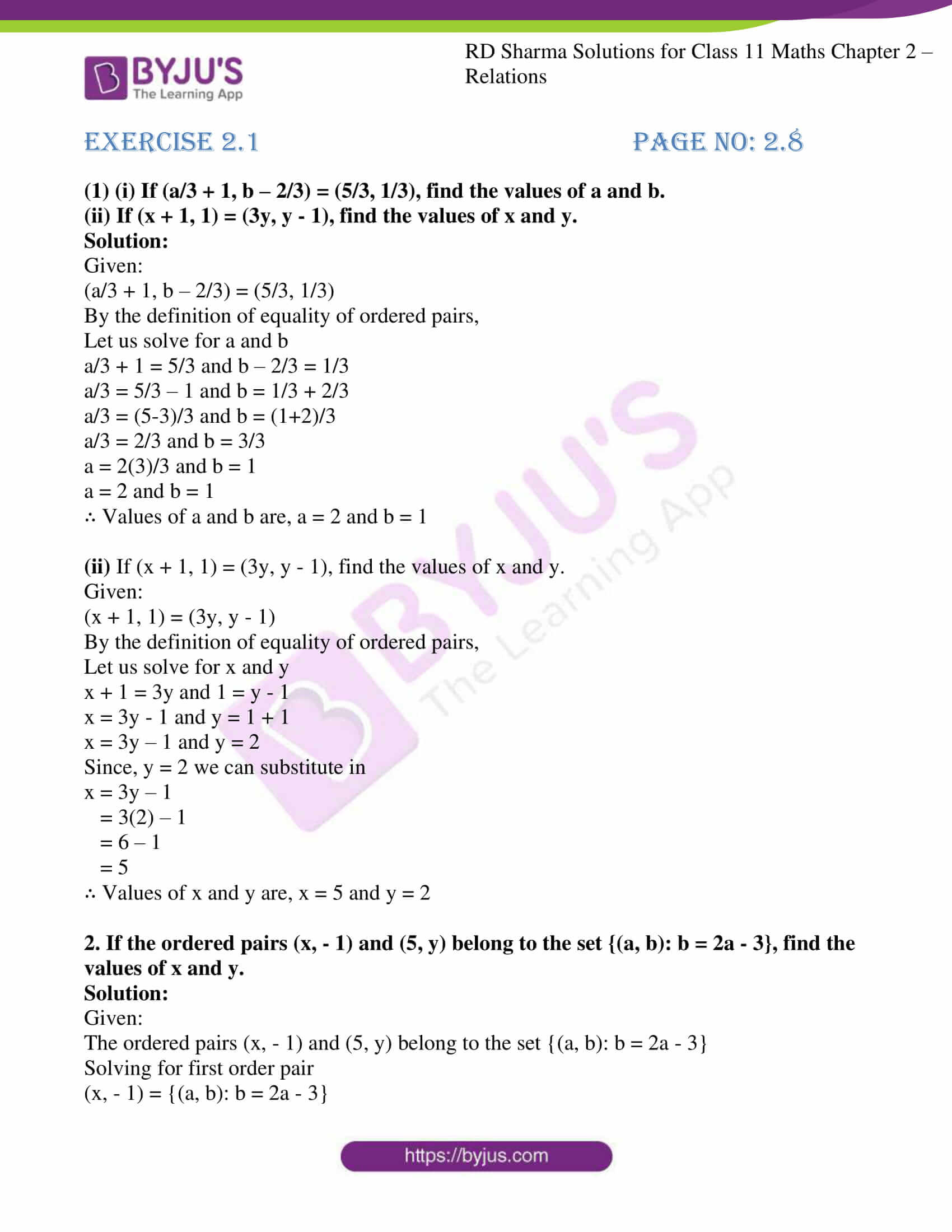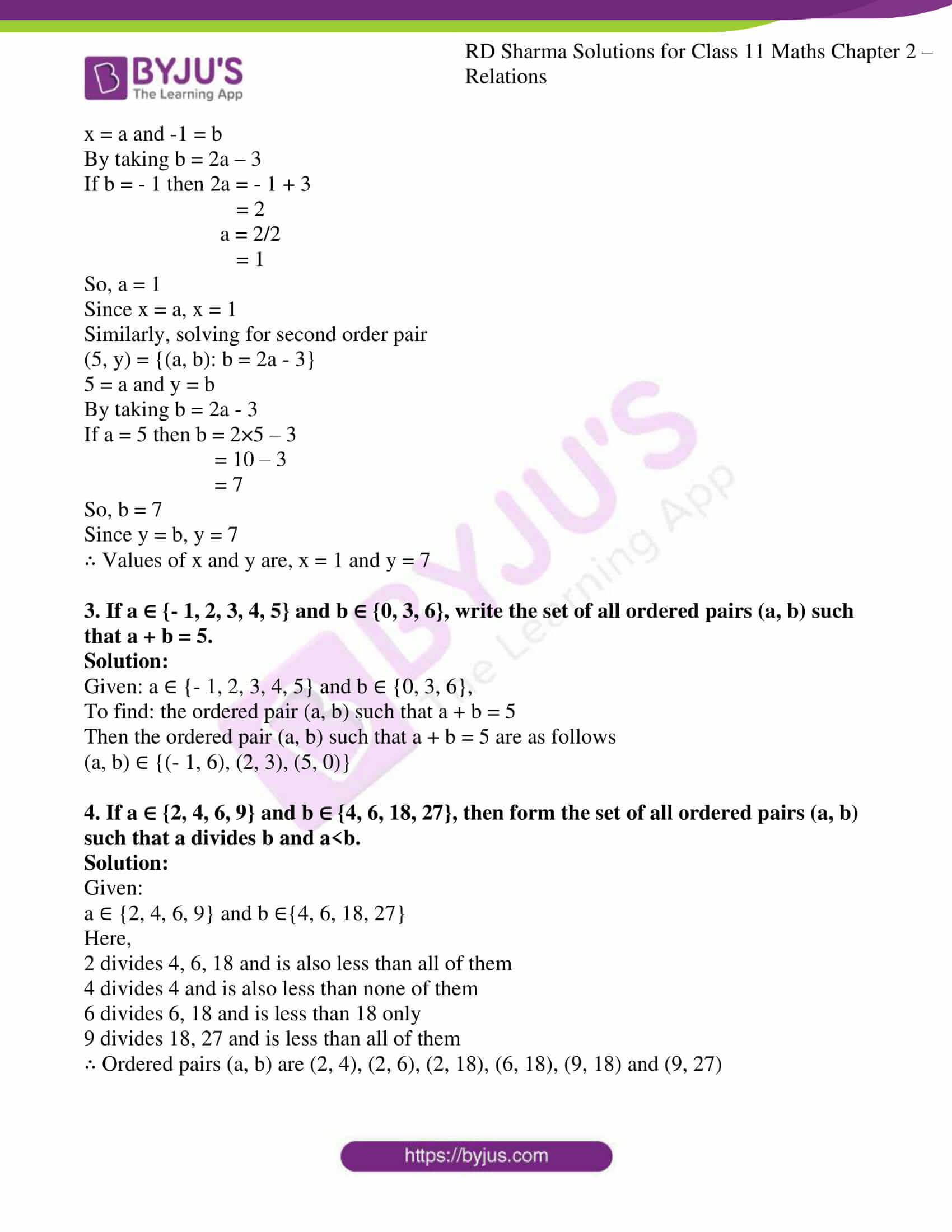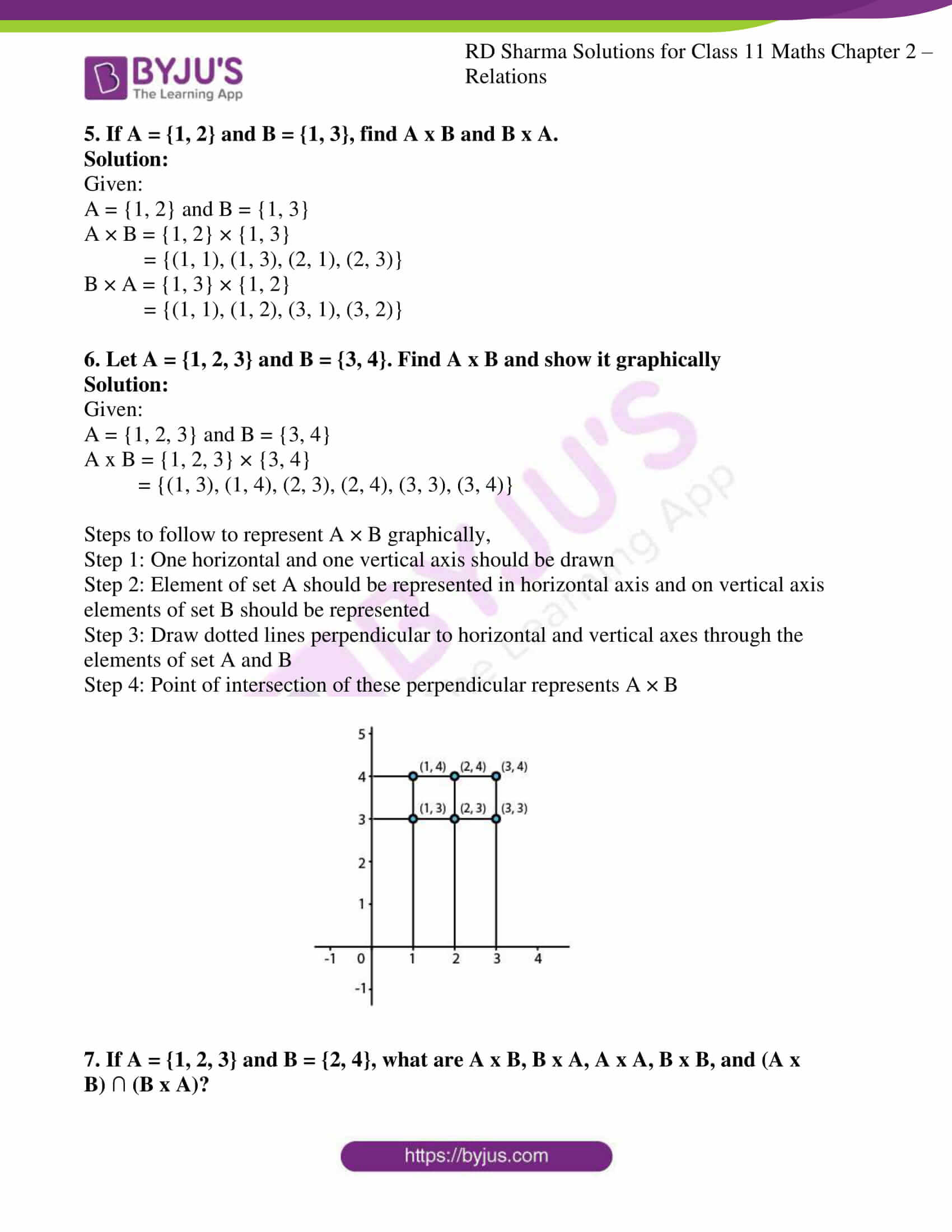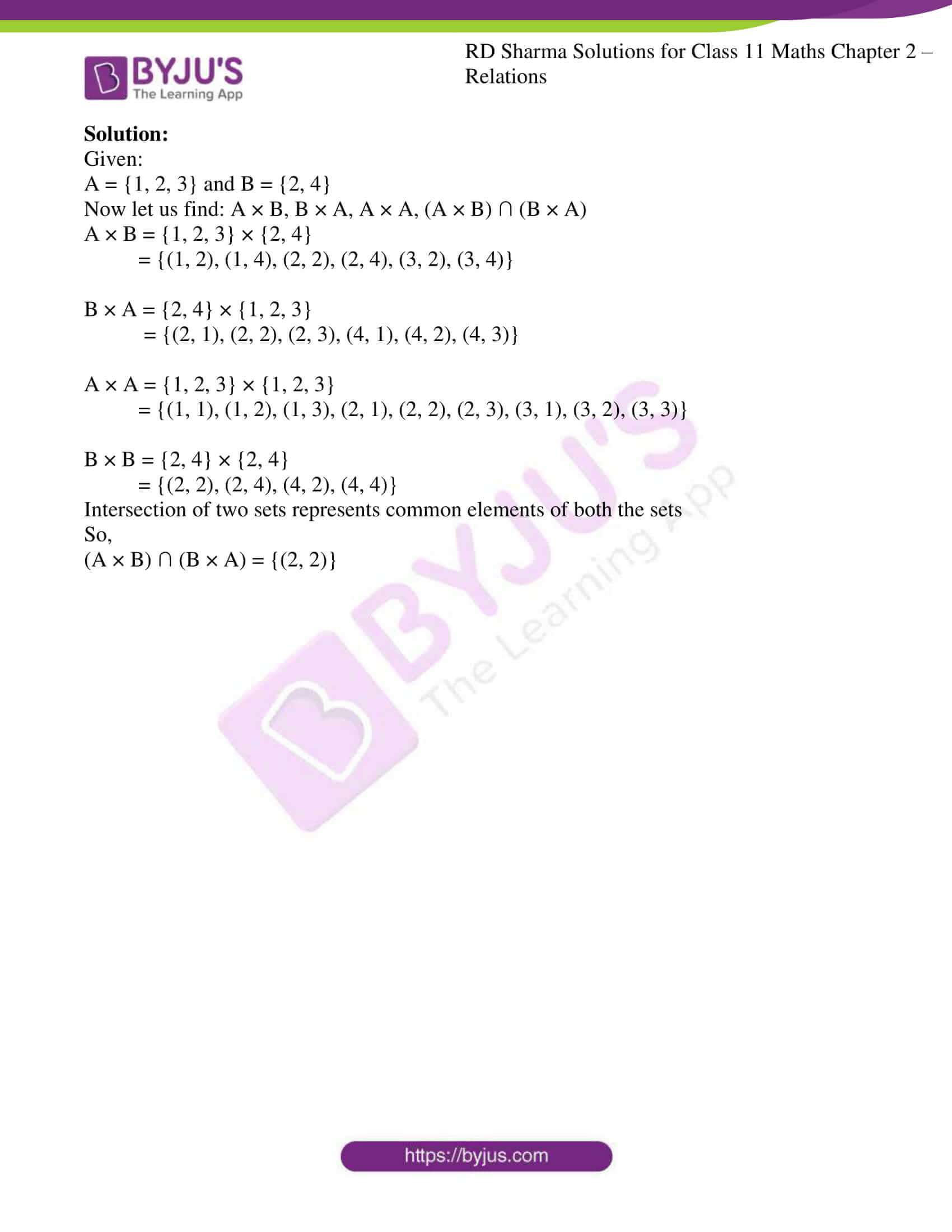### Access answers to RD Sharma Solutions for Class 11 Maths Exercise 2.1 Chapter 2 – Relations

(1) (i) If (a/3 + 1, b – 2/3) = (5/3, 1/3), find the values of a and b.

(ii) If (x + 1, 1) = (3y, y – 1), find the values of x and y.

Solution:

Given:

(a/3 + 1, b – 2/3) = (5/3, 1/3)

By the definition of equality of ordered pairs,

Let us solve for a and b

a/3 + 1 = 5/3 and b – 2/3 = 1/3

a/3 = 5/3 – 1 and b = 1/3 + 2/3

a/3 = (5-3)/3 and b = (1+2)/3

a/3 = 2/3 and b = 3/3

a = 2(3)/3 and b = 1

a = 2 and b = 1

∴ Values of a and b are, a = 2 and b = 1

(ii) If (x + 1, 1) = (3y, y – 1), find the values of x and y.

Given:

(x + 1, 1) = (3y, y – 1)

By the definition of equality of ordered pairs,

Let us solve for x and y

x + 1 = 3y and 1 = y – 1

x = 3y – 1 and y = 1 + 1

x = 3y – 1 and y = 2

Since, y = 2 we can substitute in

x = 3y – 1

= 3(2) – 1

= 6 – 1

= 5

∴ Values of x and y are, x = 5 and y = 2

2. If the ordered pairs (x, – 1) and (5, y) belong to the set {(a, b): b = 2a – 3}, find the values of x and y.

Solution:

Given:

The ordered pairs (x, – 1) and (5, y) belong to the set {(a, b): b = 2a – 3}

Solving for first order pair

(x, – 1) = {(a, b): b = 2a – 3}

x = a and -1 = b

By taking b = 2a – 3

If b = – 1 then 2a = – 1 + 3

= 2

a = 2/2

= 1

So, a = 1

Since x = a, x = 1

Similarly, solving for second order pair

(5, y) = {(a, b): b = 2a – 3}

5 = a and y = b

By taking b = 2a – 3

If a = 5 then b = 2×5 – 3

= 10 – 3

= 7

So, b = 7

Since y = b, y = 7

∴ Values of x and y are, x = 1 and y = 7

3. If a ∈ {- 1, 2, 3, 4, 5} and b ∈ {0, 3, 6}, write the set of all ordered pairs (a, b) such that a + b = 5.

Solution:

Given: a ∈ {- 1, 2, 3, 4, 5} and b ∈ {0, 3, 6},

To find: the ordered pair (a, b) such that a + b = 5

Then the ordered pair (a, b) such that a + b = 5 are as follows

(a, b) ∈ {(- 1, 6), (2, 3), (5, 0)}

4. If a ∈ {2, 4, 6, 9} and b ∈ {4, 6, 18, 27}, then form the set of all ordered pairs (a, b) such that a divides b and a<b.

Solution:

Given:

a ∈ {2, 4, 6, 9} and b ∈{4, 6, 18, 27}

Here,

2 divides 4, 6, 18 and is also less than all of them

4 divides 4 and is also less than none of them

6 divides 6, 18 and is less than 18 only

9 divides 18, 27 and is less than all of them

∴ Ordered pairs (a, b) are (2, 4), (2, 6), (2, 18), (6, 18), (9, 18) and (9, 27)

5. If A = {1, 2} and B = {1, 3}, find A x B and B x A.

Solution:

Given:

A = {1, 2} and B = {1, 3}

A × B = {1, 2} × {1, 3}

= {(1, 1), (1, 3), (2, 1), (2, 3)}

B × A = {1, 3} × {1, 2}

= {(1, 1), (1, 2), (3, 1), (3, 2)}

6. Let A = {1, 2, 3} and B = {3, 4}. Find A x B and show it graphically

Solution:

Given:

A = {1, 2, 3} and B = {3, 4}

A x B = {1, 2, 3} × {3, 4}

= {(1, 3), (1, 4), (2, 3), (2, 4), (3, 3), (3, 4)}

Steps to follow to represent A × B graphically,

Step 1: One horizontal and one vertical axis should be drawn

Step 2: Element of set A should be represented in horizontal axis and on vertical axis elements of set B should be represented

Step 3: Draw dotted lines perpendicular to horizontal and vertical axes through the elements of set A and B

Step 4: Point of intersection of these perpendicular represents A × B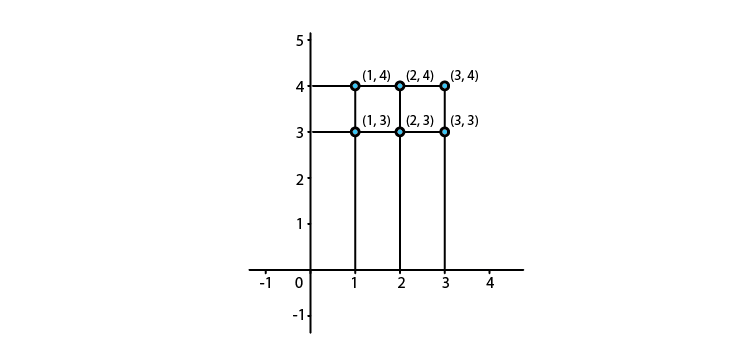7. If A = {1, 2, 3} and B = {2, 4}, what are A x B, B x A, A x A, B x B, and (A x B) ∩ (B x A)?

Solution:

Given:

A = {1, 2, 3} and B = {2, 4}

Now let us find: A × B, B × A, A × A, (A × B) ∩ (B × A)

A × B = {1, 2, 3} × {2, 4}

= {(1, 2), (1, 4), (2, 2), (2, 4), (3, 2), (3, 4)}

B × A = {2, 4} × {1, 2, 3}

= {(2, 1), (2, 2), (2, 3), (4, 1), (4, 2), (4, 3)}

A × A = {1, 2, 3} × {1, 2, 3}

= {(1, 1), (1, 2), (1, 3), (2, 1), (2, 2), (2, 3), (3, 1), (3, 2), (3, 3)}

B × B = {2, 4} × {2, 4}

= {(2, 2), (2, 4), (4, 2), (4, 4)}

Intersection of two sets represents common elements of both the sets

So,

(A × B) ∩ (B × A) = {(2, 2)}